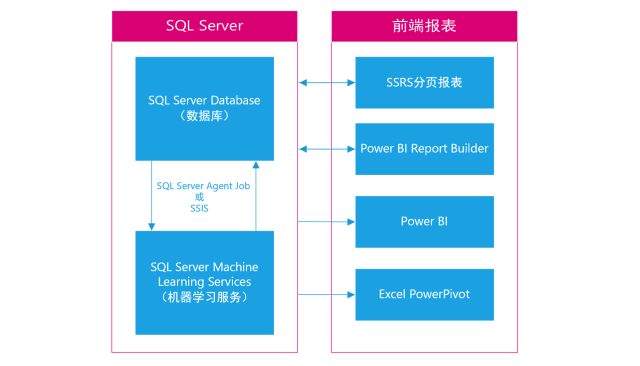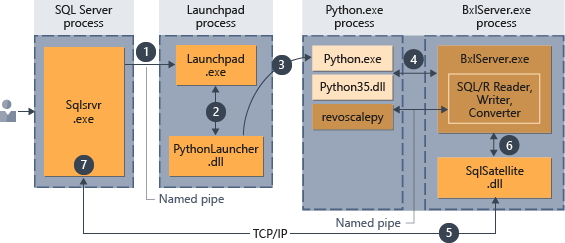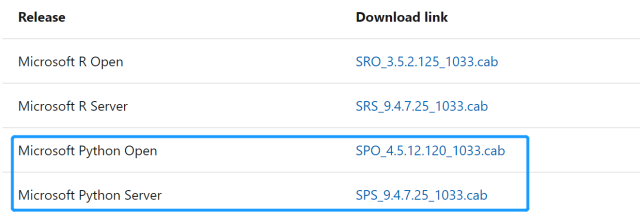# SQL Server 机器学习服务-概述与实战### 什么是机器学习服务？

（注：SQL Server 2019还提供了对Java支持，但不属于机器学习服务，在此忽略）

### 为何要使用机器学习服务？

• 可重复利用性。执行包含Python脚本的存储过程训练出的模型可以进行序列化，以单个值的形式存储在实体表中，只要模型调试好就可以在以后需要时重复利用。比如你可以提供给其他应用程序（如SSIS) 或其他的存储过程继续调用，部署到生产环境中，定期执行数据挖掘。
• 拓展性。SQL Server 机器学习服务使用Anaconda发行版，内置丰富的Python库，还可以安装第三方框架，如 TensorFlow和scikit-learn。
• 安全性。此功能在数据所在的位置运行脚本，无需通过网络将数据传输到其他服务器。通过在 SQL Server 管理的安全框架内执行受信任的脚本语言，数据库管理员可以在维持安全性的同时允许数据挖掘工程师访问企业数据。
• 可监控性。可以在SSMS中使用由微软提供或自行设计的报表监视Python的执行情况（关于安装此报表）。
• 与BI工具集成。存储过程中的参数值可以传递至Python，这意味着在使用SSRS（或Power BI Report Builder) 构建的报表中，用户可以直接调整机器学习算法中的参数，将算法模型的调优及结果的呈现自动化。比如使用多元线性回归预测销售数据，通过调整模型中不同的参数来优化模型（对于此，如果你使用Power BI, 此文提供了使用DAX建模及调优的用例）

（若针对于R语言，还有一个优势是可以以多线程的方式执行R脚本，而Python本身就支持多线程）

### 机器学习服务的运行原理（注：关于revoscalepy, 这是一个由微软开发的Python机器学习库，它提供了一些常用的机器学习算法，如决策树，线性回归以及Logistic回归等），支持分布和远程计算环境，详见文档

### 关于安装与部署

（如果你需要下载全新的SQL Server可点此到达下载页面）（注：对于简体中文版需要将CAB文件后缀名由1033改为2052，否则安装程序会找不到CAB文件从而导致报错）

### 如何使用机器学习服务训练预测模型（Python）

（提示：建议先使用Python IDE完成Pyhon脚本，一个好的建议是打开机器学习服务安装根目录，打开jupyter-notebook.exe，使用jupyter notebook测试Python脚本）

#### 1.创建一个存储过程—用于训练数据集以生成预测模型

（其中：@input_data_参数用于获取数据集）

``````DROP PROCEDURE IF EXISTS generate_model_rfc;
go
CREATE PROCEDURE generate_model_rfc (@trained_model varbinary(max) OUTPUT)
AS
BEGIN
EXECUTE sp_execute_external_script
@language = N'Python'
, @script = N'
import numpy as np
import pandas as pd
import pickle
from sklearn.model_selection import train_test_split
from sklearn.ensemble import RandomForestClassifier
from sklearn import metrics
dt = train_data
dt["Level"] = dt["Level"].astype(''category'')
X = dt[["Fixed Acidity","Volatile Acidity","Citric acid","Residual Sugar","Chlorides","Free Sulfur Dioxide","Total Sulfur Dioxide","Density","PH","Sulphates","Alcohol"]]
Y = dt[["Level"]]
X_Train, X_Test, Y_Train, Y_Test = train_test_split(X, Y, test_size=0.3, random_state=42)
RFC=RandomForestClassifier(n_estimators=100,criterion=''gini'',min_samples_split=2,max_depth=2)
RFC.fit(X_Train,Y_Train.values.ravel())
trained_model = pickle.dumps(RFC)
'
, @input_data_1 = N'select "Fixed Acidity", "Volatile Acidity", "Citric acid", "Residual Sugar", "Chlorides", "Free Sulfur Dioxide", "Total Sulfur Dioxide", "Density", "PH", "Sulphates", "Alcohol", "Level" from dbo.RedWineQuality'
, @input_data_1_name = N'train_data'
, @params = N'@trained_model varbinary(max) OUTPUT'
, @trained_model = @trained_model OUTPUT;
END;
GO
``````

#### 2.创建一个表–用于存储预测模型

``````CREATE TABLE dbo.my_py_models (
model_name VARCHAR(50) NOT NULL DEFAULT('default model') PRIMARY KEY,
model_object VARBINARY(MAX) NOT NULL
);
GO
``````

#### 3.执行第一个存储过程–生成预测模型并存储在上述创建的表(my_py_models)中

``````DECLARE @model_object VARBINARY(MAX);
EXEC generate_model_rfc @model_object OUTPUT;
INSERT INTO my_py_models (model_name, model_object) VALUES('RandomForestClassification(RFC)', @model_object);
``````

#### 4.创建第二个存储过程–用于调用现有预测模型对新增数据进行预测

``````DROP PROCEDURE IF EXISTS py_predict_rfc;
GO
CREATE PROCEDURE py_predict_rfc (@model varchar(100))
AS
BEGIN
DECLARE @py_model varbinary(max) = (select model_object from dbo.my_py_models where model_name = @model);
EXEC sp_execute_external_script
@language = N'Python',
@script = N'
import pickle
import numpy as np
import pandas as pd
from sklearn.model_selection import train_test_split
dt = data
dt["Level"] = dt["Level"].astype(''category'')
X = dt[["Fixed Acidity","Volatile Acidity","Citric acid","Residual Sugar","Chlorides","Free Sulfur Dioxide","Total Sulfur Dioxide","Density","PH","Sulphates","Alcohol"]]
Y = dt[["Level"]]
X_Train, X_Test, Y_Train, Y_Test = train_test_split(X, Y, test_size=0.3, random_state=42)
Predictions = py_model_rfc.predict(X_Test)
OutputDataSet=pd.DataFrame(data=Predictions,columns=[''Prediction''])
OutputDataSet[''Actual''] = pd.Series(Y_Test[''Level''].values,index=np.arange(0,len(Y_Test)))
'
, @input_data_1 = N'select "Fixed Acidity", "Volatile Acidity", "Citric acid", "Residual Sugar", "Chlorides", "Free Sulfur Dioxide", "Total Sulfur Dioxide", "Density", "PH", "Sulphates", "Alcohol", "Level" from dbo.RedWineQuality'
, @input_data_1_name = N'data'
, @params = N'@py_model varbinary(max)'
, @py_model = @py_model
with result sets (("Prediction" int, "Actual" int));
END;
GO
``````

#### 5.创建一个新表–用于存储模型测试结果

``````CREATE TABLE [dbo].[py_rfc_predictions](
[Prediction] int,
[Actual] int
)
``````

#### 6.执行第二个存储过程–预测数据并将结果输出到上述新表(py_rfc_predictions)中

``````INSERT INTO py_rfc_predictions
EXEC py_predict_rfc 'RandomForestClassification(RFC)';
SELECT * FROM py_rfc_predictions;
``````

### 总结

SQL Server和Python的强强联手，能够使报表开发者更好地获取到经过机器学习算法生成的深度挖掘的数据，这类数据往往难以使用前端的BI工具完成，比如在Power BI中，利用DAX只能实现简单基础的算法（如用DAX实现KNN分类算法），在Power BI Premium以及Power BI Embedded中虽然具备机器学习功能但只支持云端版本，且这种机器学习模型的训练相比用户自己根据自身需求直接使用Python代码训练的方式而言，无论是可选择性还是效果等等，都有很明显的差距。显然在企业环境中，使用机器学习服务挖掘数据并存储在数据库，然后使用前端BI工具直接读取结果集是更规范更实用的办法。